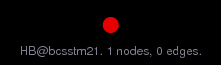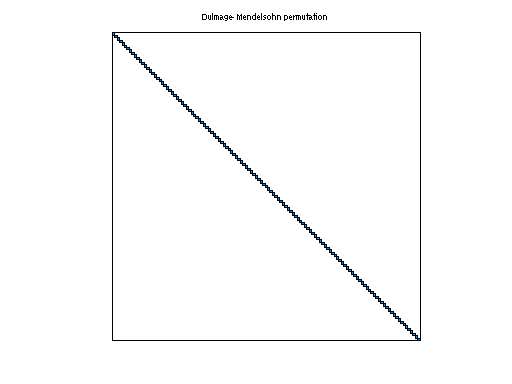Matrix: HB/bcsstm21

Description: SYMMETRIC MASS MATRIX - CLAMPED SQUARE PLATE(undirected graph drawing)• Matrix group: HB
• download as a MATLAB mat-file, file size: 11 KB. Use UFget(71) or UFget('HB/bcsstm21') in MATLAB.

 Matrix properties number of rows 3,600 number of columns 3,600 nonzeros 3,600 structural full rank? yes structural rank 3,600 # of blocks from dmperm 3,600 # strongly connected comp. 3,600 explicit zero entries 0 nonzero pattern symmetry symmetric numeric value symmetry symmetric type real structure symmetric Cholesky candidate? yes positive definite? yes

 author J. Lewis editor I. Duff, R. Grimes, J. Lewis date 1984 kind structural problem 2D/3D problem? yes

 Ordering statistics: result nnz(chol(P*(A+A'+s*I)*P')) with AMD 3,600 Cholesky flop count 3.6e+03 nnz(L+U), no partial pivoting, with AMD 3,600 nnz(V) for QR, upper bound nnz(L) for LU, with COLAMD 3,600 nnz(R) for QR, upper bound nnz(U) for LU, with COLAMD 3,600

 SVD-based statistics: norm(A) 0.000146606 min(svd(A)) 6.18187e-06 cond(A) 23.7154 rank(A) 3,600 sprank(A)-rank(A) 0 null space dimension 0 full numerical rank? yes

 singular values (MAT file): click here SVD method used: s = svd (full (A)) ; status: ok